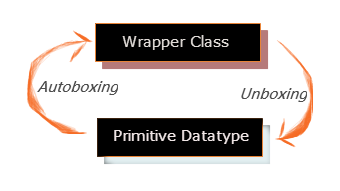Showing posts with label Unboxing. Show all posts
Showing posts with label Unboxing. Show all posts## Boxing and Unboxing

Boxing and Unboxing in java is nothing but conversion from Wrapper classes to primitive datatypes and vice-verse in Java. In our earlier tutorial we have seen about list of Wrapper classes and their corresponding primitive datatypes in Java
In that tutorial already we have seen about 8 wrapper classes and their class hierarchy also. So what is Boxing and Unboxing is when we convert an int to an Integer, a double to a Double etc., is called as Boxing and opposite conversion like Integer to int, Double to double is called as Unboxing. Now lets see simple example of both Boxing and Unboxing in java.

```public class BoxingTest {

public static void main(String[] args) {

int _int = 1;
byte _byte = 2;
short _short = 3;
long _long = 4;
float _float = 5.5f;
double _double = 6;
char _char = 'a';
boolean _boolean = true;

// Boxing (Primitive datatype to Wrapper classes)
Integer w_int = new Integer(_int);
Byte w_byte = new Byte(_byte);
Short w_short = new Short(_short);
Long w_long = new Long(_long);
Float w_float = new Float(_float);
Double w_double = new Double(_double);
Character w_char = new Character(_char);
Boolean w_boolean = new Boolean(_boolean);

// Unboxing (Wrapper class to Primitive datatypes)
int _int1 = w_int;
byte _byte1 = w_byte;
short _short1 = w_short;
long _long1 = w_long;
float _float1 = w_float;
double _double1 = w_double;
char _char1 = w_char;
boolean _boolean1 = w_boolean;
}
}
```

In above example we have seen how converting primitive datatype to Wrapper class values is called as Boxing and next same Wrapper class object are stored into Primitive datatype is called as unboxing in java.